PHY294H - Lecture 37

Two views of light

Light as a wave - From Maxwell's equations

Linearly polarized (with the electric field in the x-direciton) EM waves moving through free space are described by,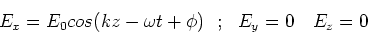(1)

and,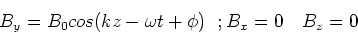(2)

They travel with speed c which is given by,(3)

The amplitudes of the waves are related by,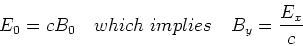(4)

The wave velocity of the wave is, so we have,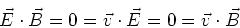(5)

This wave theory of light can explain many of the phenomena that we shall study, including reflection, refraction, interference and diffraction.

Note that if light travels in matter, its speed changes to,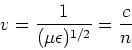(6)

where n is the refrective index of light in materials and it is related to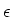and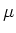by,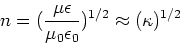(7)

The latter expression is true as most optical material have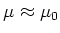. Often the refractive index of a material depends on the light frequency, in which case the material is dispersive''.

Light as a particle - from quantum mechanics and relativity

Two key equations that we need are from modern physics. Planck showed that light comes in bundles of energy called photons, where the energy of a photon is related to its frequency, f, by,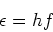(8)

where h is a Planck's constant and is very small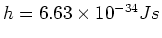. Einstein then showed that massless particles carry momentum. For massless particles, energy and momentum are related by,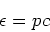(9)

where c is the speed of light. De Broglie argued that all particles have a wavelength which is related to their momentum via the relation,(10)

It is easy to see that Planck's law and Einstein's equation lead to de Broglie's equation for massless particles. The particle nature of light is evident at small distances, short times and/or at high energies. For example, spectroscopy and laser phenomena rely on the quantum nature of light, as does high energy scattering (e.g. Compton scattering) and the photo-electric effect.

Note that many of the experiments with light may be interpretted using either the wave theory or particle (quantum) theory. This was the origin of many controversies concerning the theory of light in the 19th and early 20th centuries. Of course the full quantum theory of light contains the wave picture in the classical limit, which is a delight to be anticipated in higher level physics courses.

Important properties of light

From the structure of EM waves, we will now understand the energy density, the energy flux, the momentum and the polarization properties of these waves.

Energy density u

First let's look at the energy density, u, of an EM wave. This is easy to write down as we already know the energy density of electric and magnetic fields, so we have,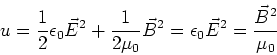(11)

The average(root mean square) energy density in the electric and magnetic fields is then given by,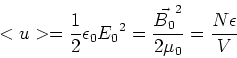(12)

Energy flux (power) and Poynting vector,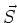Water waves and waves on strings carry energy. EM waves do too. Oscillating electric and magnetic fields can lead to motion of charges which is the way in which EM waves transfer their energy to matter. The amount of energy which is available per unit time per unit area is called the energy flux. We write,

 dU = A c u dt (13)

From which we find that the magnitude of the energy flux is ,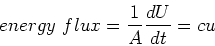(14)

This expression can be found from the cross product,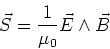(15)is called the Poynting vector and has the advantage that it also tells us the direction of the energy flux (in the direction of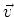). From the expressions for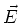andit is evident that,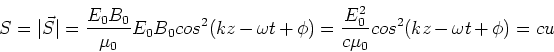(16)

In many calculations, it is the time averaged value of S that is important. This is called the intensity, that is I = <S>, which is given by,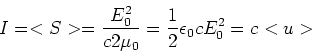(17)

Momentum density

Water waves and waves on strings have momentum. Their momentum is due to the motion of the media in which they travel. EM waves also have momentum. This is harder to see classically, however from the quantum viewpoint it is evident. De Broglie's relation iswhich relates the momentum to the wavelength of a wave and to Planck's constant h. Nevertheless it is possible to deduce the momentum of an EM wave from classical arguments. This is evident upon placing a positive charge in the path of the EM wave. When the electric field is in the positive x direction, it accelerates the charge in that direction. This charge moves in the presence of the magnetic field which is in the y-direction, The magnetic force on this moving charge is in the z direction, that is, in the direction of motion of the wave. The wave thus pushes the charge in the direction of motion of the wave. Momentum is thus transferred from the EM wave to the charged particle. It is possible to derive the relation we need from this argument.

However, the most natural way to find the relation between the energy density of the wave and the momentum density of the wave is to use the particulate nature of the light. Using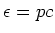, the momentum density is given by,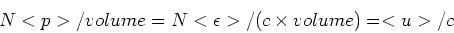(18)

This implies that the momentum density in the wave is u/c.

Photons may be reflected or absorbed. Since photons carry momentum, these events lead to pressure at the macroscopic scale. This pressure is called radiation pressure and is the pressure which stops the sun from collapsing. Consider a flat surface. Photons which hit the flat surface may reflect or absorb. Let's assume normal incidence, that is their velocity is normal to the surface. If they reflect elastically from the surface, a momentum of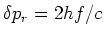is transferred to the surface, while if they are absorbed by the surface, a momentum of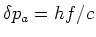is transferred to the surface. In general there is partial relection and partial absorption, in which case we have,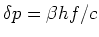, with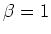for absorption and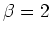for reflection, for normal incidence. Clearly reflection is more effective at transferring momentum. The pressure is the force per unit area p = F/A. The force is the rate of change of momentum which is the change in momentum due to one photon hitting the surface times the number hitting the surface per unit time, that is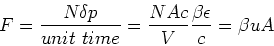(19)

The radiation pressure is then equivalent to the energy density times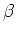, that is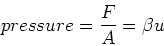(20)

The intensity is the average power per unit area and for a light wave is given by,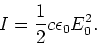(21)

It is important to know how the intensity of a wave decreases as a function of distance from the source. If we assume a point source of energy, then the intensity decreases as 1/R2. If we assume a dipole source there is also an angular dependence, which is given by,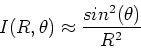(22)

Geometrical optics - reflection and refraction

In many cases, the behavior of light can be understood by using rays to trace the way in which a beam of light reflects from surfaces and the way in which a beam of light refracts when passing through a surface. Mirrors, lenses, telescopes and microscopes can all be understood using the simple principles of geometrical optics. There are two basic laws of geometrical optics which describe the way in which light rays behaves at a surface,

Reflection law

The angle of incidence is equal to the angle of reflection,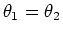.

Refraction law (Snell's law)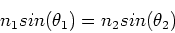(23)

Note: Angles are measured with respect to the normal to the surface.